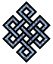fail2ban bad ip database: ip 103.241.146.65

| ip database | live view | stats | report | help | api key:

 ip: 103.241.146.65 hostname: static-103-241-146-65.ctrls.in country:[IN] India first reported: 20.11.2018 21:35.00 GMT+0200 last reported: 27.02.2019 22:52.45 GMT+0200 time period: 99d 01h 17m 45s total reports: 12 reported by: 4 host(s) filter(s): ssh (11) ssh (1) tor exit node no badips.com db Lookupport scan of '103.241.146.65':

[-hide]
```# Nmap 6.40 scan initiated Tue Nov 20 21:35:02 2018 as: /usr/bin/nmap -sU -sS -O 103.241.146.65
Nmap scan report for static-103-241-146-65.ctrls.in (103.241.146.65)
Host is up (0.12s latency).
Not shown: 998 closed ports, 991 open|filtered ports
PORT     STATE    SERVICE
22/tcp   open     ssh
25/tcp   open     smtp
80/tcp   open     http
110/tcp  open     pop3
135/tcp  filtered msrpc
139/tcp  filtered netbios-ssn
143/tcp  open     imap
443/tcp  open     https
445/tcp  filtered microsoft-ds
1723/tcp open     pptp
8080/tcp open     http-proxy
No exact OS matches for host (If you know what OS is running on it, see http://nmap.org/submit/ ).
TCP/IP fingerprint:
OS:SCAN(V=6.40%E=4%D=11/20%OT=22%CT=1%CU=2160%PV=N%G=Y%TM=5BF471F6%P=x86_64
OS:-pc-linux-gnu)SEQ(SP=105%GCD=1%ISR=10B%TI=Z%TS=8)OPS(O1=M5B4ST11NW7%O2=M
OS:5B4ST11NW7%O3=M5B4NNT11NW7%O4=M5B4ST11NW7%O5=M5B4ST11NW7%O6=M5B4ST11)WIN
OS:(W1=7120%W2=7120%W3=7120%W4=7120%W5=7120%W6=7120)ECN(R=Y%DF=Y%TG=40%W=72
OS:10%O=M5B4NNSNW7%CC=Y%Q=)T1(R=N)T1(R=Y%DF=Y%TG=40%S=O%A=S+%F=AS%RD=0%Q=)T
OS:2(R=N)T3(R=N)T4(R=N)T5(R=N)T5(R=Y%DF=Y%TG=40%W=0%S=Z%A=S+%F=AR%O=%RD=0%Q
OS:=)T6(R=N)T7(R=N)U1(R=N)IE(R=Y%DFI=N%TG=40%CD=S)

OS detection performed. Please report any incorrect results at http://nmap.org/submit/ .
# Nmap done at Tue Nov 20 21:43:34 2018 -- 1 IP address (1 host up) scanned in 512.92 seconds
```
```Σ = 74 | Δt = 0.0039288997650146s
```